# Digital Logic - Combinational Circuits

56:

What logic function is performed by the circuit shown below?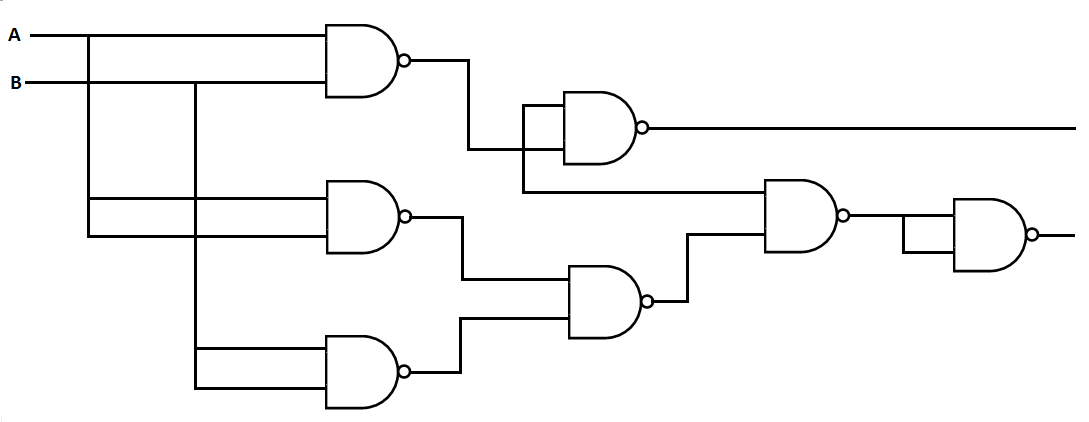A. Ring counter B. Ripple counter C. Full adder D. Half adder Answer Report Discuss Option: D Explanation : Here total 7 NAND gates are present  and in half adder one AND and one EX-OR gate are required. In turn  one EX-OR gate can be built by 5 NAND where as one AND gate can be built by 2 NAND  gates so this is  diagram of half adder. So the answer is (D). Click on Discuss to view users comments. wdw said: (8:41pm on Sunday 5th January 2014) Option D is Correct Write your comments here:
57:

What is the Boolean expression for the following circuit?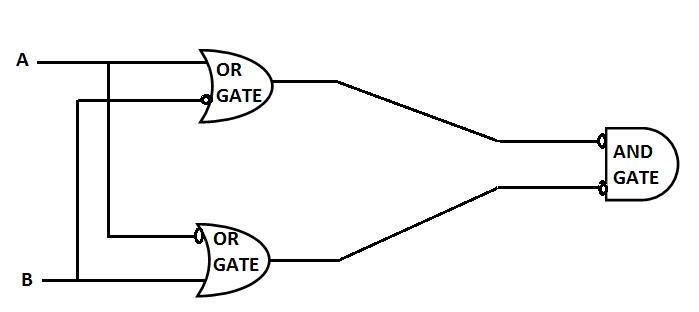A. F(A, B) = ( A + B' )' . ( B + A' )' B. F( A, B ) = 1 ( Tautology ) C. F( A, B ) = ⊕ ( inconsistency) D. F ( A, B ) = A ⊕  B ( A exclusive OR'ed with B) Answer Report Discuss Option: A Explanation :  Ans is  A as  input to 1st OR gate is A, and B' so output   of 1st OR gate is (A+B') In the same way output  for 2nd OR gate is (A'+B) So Final output will be (A+B')'. (A'+B)' Click on Discuss to view users comments. Write your comments here:
58:

In the circuit shown below, which logic function does this circuit generate?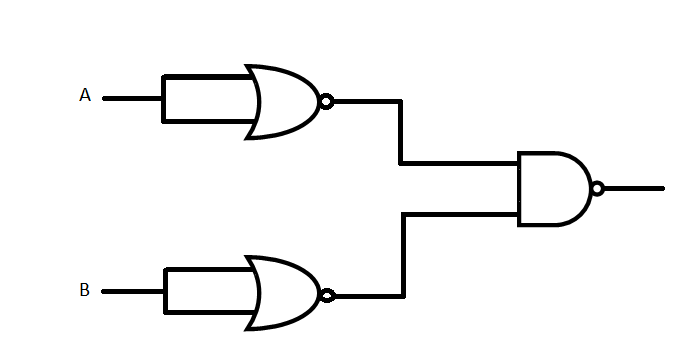A. AND B. NOR C. NAND D. OR Answer Report Discuss Option: D Explanation : Click on Discuss to view users comments. Write your comments here:
59:

Output of the following circuit is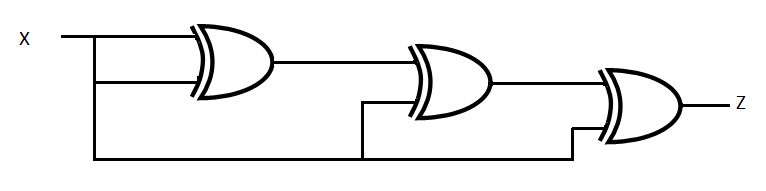A. 0 B. 1 C. x D. x' Answer Report Discuss Option: A Explanation : Truth Table for XOR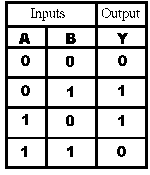Click on Discuss to view users comments. Write your comments here:
60:

A small dot or circle printed on top of an IC indicates

 A. Vcc B. Gnd C. Pin 14 D. Pin 1 Answer Report Discuss Option: D Explanation : Click on Discuss to view users comments. Write your comments here: